Reach Us+44-1474-556909
A Numericandndash;Analytic Method for Fractional Order Nonlinear PDEandrsquo;s With Modified Riemann-Liouville Derivative by Means of Fractional Variational Iteration Method
Journal of Applied & Computational Mathematics
All submissions of the EM system will be redirected to Online Manuscript Submission System. Authors are requested to submit articles directly to Online Manuscript Submission System of respective journal.

# A Numeric–Analytic Method for Fractional Order Nonlinear PDE’s With Modified Riemann-Liouville Derivative by Means of Fractional Variational Iteration Method

Mehmet Merdan*

Gümüshane University, Department of Mathematics Engineering, 29100-Gümüshane, Turkey

*Corresponding Author:
Mehmet Merdan
Gümüshane University
Department of Mathematics Engineering
29100-Gümüshane, Turkey
E-mail: [email protected]

Received Date: May 16, 2012; Accepted Date: June 11, 2012; Published Date: June 15, 2012

Citation: Merdan M (2012) A Numeric–Analytic Method for Fractional Order Nonlinear PDE’s With Modified Riemann-Liouville Derivative by Means of Fractional Variational Iteration Method. J Appl Computat Math 1:113. doi:10.4172/2168-9679.1000113

Copyright: © 2012 Merdan M. This is an open-access article distributed under the terms of the Creative Commons Attribution License, which permits unrestricted use, distribution, and reproduction in any medium, provided the original author and source are credited.

Visit for more related articles at Journal of Applied & Computational Mathematics

#### Abstract

In this article, an approximate analytical solution of fractional order nonlinear PDE’s with modified Riemann- Liouville derivative was obtained with the help of fractional variational iteration method (FVIM). It is showed that the solutions obtained by the FVIM are reliable and effective method for strongly nonlinear partial equations with modified Riemann-Liouville derivative. The solutions of our model equation can also be obtained from the known forms of the series solutions.

#### Keywords

Fractional variational iteration method; Fractional KDV; K (2, 2) and mKDV equation; Riemann-Liouville derivative; Burgers equation; Klein-Gordon equation

#### Introduction

It is known that various problems in electrical networks, control theory of dynamical systems, probability and statistics, electrochemistry of corrosion, chemical physics, optics, engineering, acoustics, material science and signal processing can be successfully modeled by linear or nonlinear fractional order differential equations. Methods of solutions of problems for fractional differential equations have been studied extensively by many researchers [1-8].

The variational iteration method (VIM), which proposed by Ji- Huan He , was successfully applied to autonomous ordinary and partial differential equations and other fields. Ji-Huan He  was the first to apply the variational iteration method to fractional differential equations.

Fractional convection–diffusion equation with nonlinear source term solved by Momani and Yildirim , space–time fractional advection–dispersion equation by Yildirim and Kocak , fractional Zakharov–Kuznetsov equations by Yildirim and Gulkanat , integro-differential equation by El-Shahed , non-Newtonian flow by Siddiqui et al. , fractional PDEs in fluid mechanics by Yildirim , fractional Schrödinger equation [17,18]. Different fractional partial differential equations have been studied and solved including the space-time fractional diffusion-wave equation [19-21], the fractional advection-dispersion equation [22,23], the fractional telegraph equation , the fractional KdV equation  and the linear inhomogeneous fractional partial differential equations . Recently, a new modified Riemann-Liouville left derivative is suggested by G. Jumarie [27-31]. The most recently, a new application of Fractional Variational Iteration Method (FVIM) for solving non-linear fractional coupled- KDV equations with modified Riemann-Liouville derivative performed by Merdan et al. . Roul  applied the fractional VIM for obtaining the exact and approximate analytical solutions of time fractional biological population models.

The aim of this paper is to extend the application of the variational iteration method method to solve fractional KDV, K(2,2), mKDV equation and some fractional partial equations in fluid mechanics with modified Riemann-Liouville derivative.

This paper is organized as follows:

In Basic definitions section, we gave definitions related to the fractional calculus theory briefly. In Fractional variational iteration method section, we define the solution procedure of the fractional variational iteration method. To show in efficiency of this method, we give the implementation of the FVIM for the fractional KDV, K (2,2), mKDV equation and some fractional partial equations in fluid mechanics with modified Riemann-Liouville derivative and numerical results in Applications section. The conclusions are then given at the last.

#### Basic Definitions

Here, we give some basic definitions and properties of fractional calculus theory which will be used in this paper [1,2,27-37].

Definition 1

Fractional derivative is defined as the following limit form [27-29]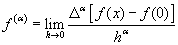(1)

This definition is close to the standard definition of derivatives (calculus for beginners), and as a direct result, the α th derivative of a constant, 0 <α <1, is zero.

Definition 2

The left-sided Riemann-Liouville fractional integral operator of order α ≥ 0, of a function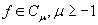is defined as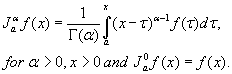(2)

The properties of the operator Jα can be found in [1,2,34].

Definition 3

The modified Riemann-Liouville derivative [27-29] is defined as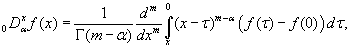(3)

where x∈[0,1], m−1≤α < m and m ≥1.

Definition 4

Fractional derivative of compounded functions [27-29] is defined as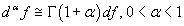(4)

Definition 5

The integral with respect to (dx)α [27-29] is defined as the solution of the fractional differential equation(5)

Lemma 1

Let f (x) denote a continuous function [27-29] then the solution of the Eq. (5) is defined as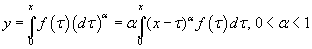(6)

For example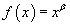in Eq. (6) one obtains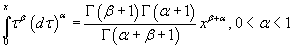(7)

Definition 6

Assume that the continuous function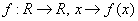has a fractional derivative of order kα , for any positive integer k and any α , 0 <α ≤1 ; then the following

equality holds, which is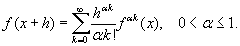(8)

On making the substitution h→ x and x→0 we obtain the fractional Mc-Laurin series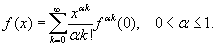(9)

#### Fractional Variational Iteration Method

To describe the solution procedure of the fractional variational iteration method, we consider the following fractional differential equation :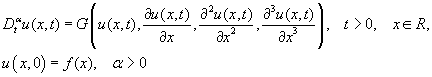(10)

According to the VIM, we can build a correct functional for Eq. (4) as follows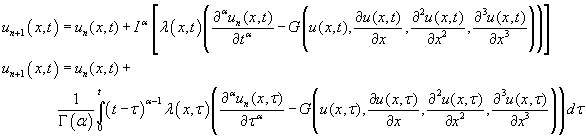(11)

Using Eq. (5), we obtain a new correction functional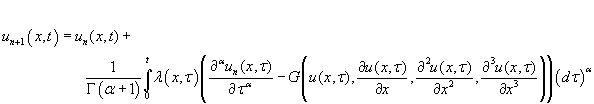(12)

It is obvious that the sequential approximations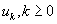can be established by determining λ , a general Lagrange’s multiplier, which can be identified optimally with the variational theory. The function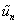is a restricted variation which means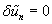. Therefore, we first designate the Lagrange multiplierλ that will be identified optimally via integration by parts. The successive approximations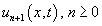of the solution u (x,t )will be readily obtained upon using the obtained Lagrange multiplier and by using any selective function u0. The initial values are usually used for choosing the zeroth approximation u0 . With λ determined, then several approximationsfollows immediately . Consequently, the exact solution may be procured by using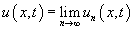(13)

#### Applications

In this section, we present the solution of nonlinear fractional partial differential equations as the applicability of FVIM.

Example 1

Consider the fractional KDV equation where 0 <α ≤1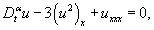(14)

With initial conditions

u (x,0) = 6x, . (15)

Construction the following functional: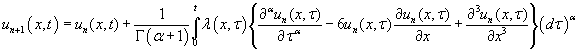(16)

We have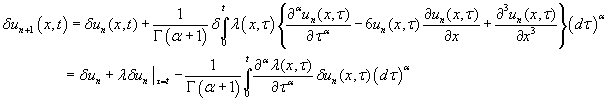(17)

Similarly, we can get the coefficients of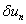to zero: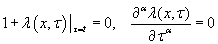(18)

The generalized Lagrange multiplier can be identified by the above equations,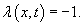(19)

Substituting Eq. (19) into Eq. (16) produces the iteration formulation as follows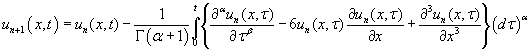(20)

Taking the initial value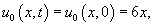we can derive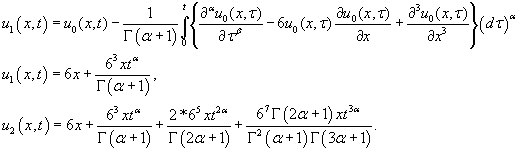(21)

For the special case α =1 is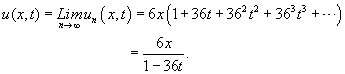(22)

Example 2

Consider the fractional K (2,2) equation where 0 <α ≤1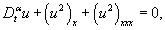(23)

With initial conditions

u (x,0) = x, . (24)

Construction the following functional: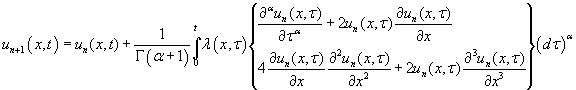(25)

We have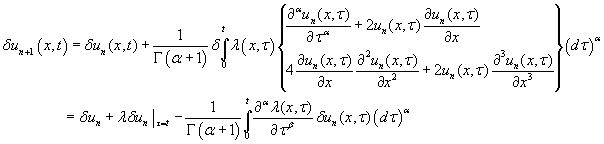(26)

Similarly, we can get the coefficients ofto zero:(27)

The generalized Lagrange multiplier can be identified by the above equations,(28)

Substituting Eq. (28) into Eq. (25) produces the iteration formulation as follows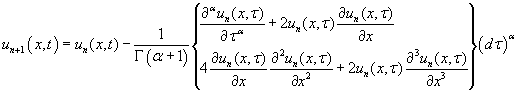(29)

Taking the initial value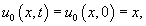we can derive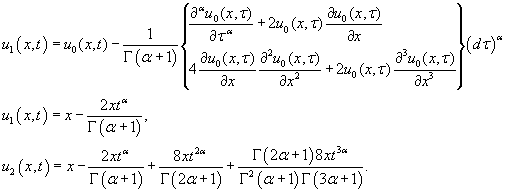(30)

For the special case α =1 is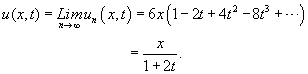(31)

Example 3

Consider the modified fractional KDV (mKDV) equation where 0 <α ≤1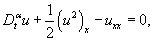(32)

With initial conditions

u (x,0) = x, . (33)

Construction the following functional: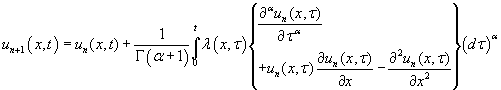(34)

We have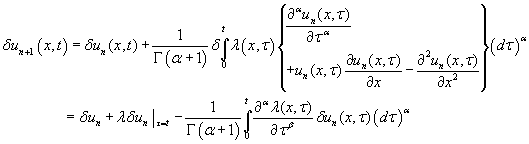(35)

Similarly, we can get the coefficients ofto zero:(36)

The generalized Lagrange multiplier can be identified by the above equations,(37)

Substituting Eq. (37) into Eq. (34) produces the iteration formulation as follows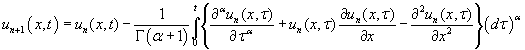(38)

Taking the initial valuewe can derive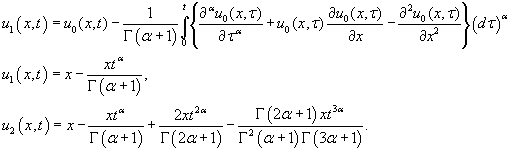(39)

For the special case α =1 is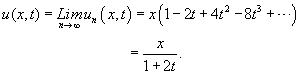(40)

Example 4

In this example we consider one–dimensional linear inhomogeneous fractional Burgers equation  where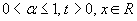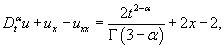(41)

With initial conditions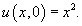(42)

Construction the following functional: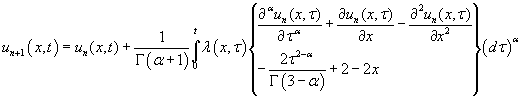(43)

We have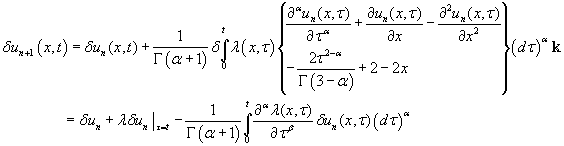(44)

Similarly, we can get the coefficients ofto zero:(45)

The generalized Lagrange multiplier can be identified by the above equations,(46)

substituting Eq. (46) into Eq. (43) produces the iteration formulation as follows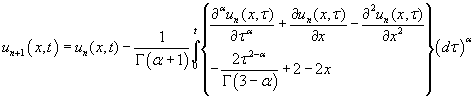(47)

Taking the initial value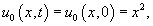we can derive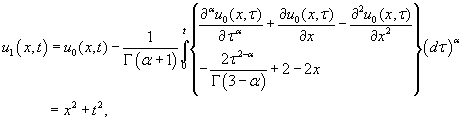(48)

By the similar operations, we have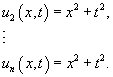(49)

Thus, the solution can be given a compact form: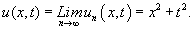(50)

Which is the exact solution of Eq.(41) and in concordance with the conditions in Eq.(42).

Example 5

Consider the following one –dimensional linear inhomogeneous fractional wave equation  where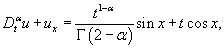(51)

With initial conditions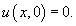(52)

Construction the following functional: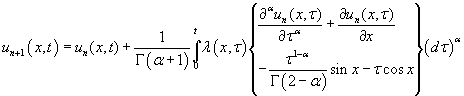(53)

We have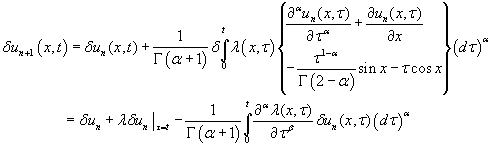(54)

Similarly, we can get the coefficients ofto zero:(55)

The generalized Lagrange multiplier can be identified by the above equations,(56)

Substituting Eq. (56) into Eq. (53) produces the iteration formulation as follows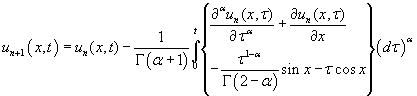(57)

Taking the initial value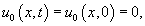we can derive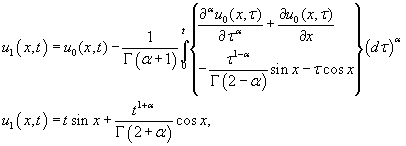(58)

By the similar operations, we have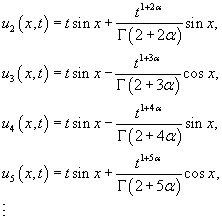(59)

Canceling the noise terms and keeping the non-noise terms yield the exact solution of Eq. (51) given by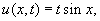(60)

Which is easily confirmed. This formally proved right in .

Example 6

We consider the following one –dimensional linear inhomogeneous fractional Klein-Gordon equation  where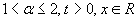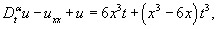(61)

With initial conditions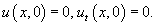(62)

Construction the following functional: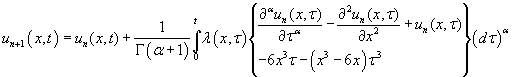(63)

We have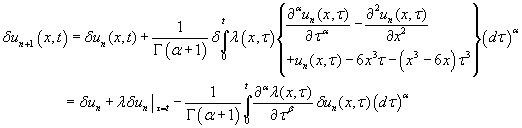(64)

Similarly, we can get the coefficients ofto zero:(65)

The generalized Lagrange multiplier can be identified by the above equations,(66)

substituting Eq. (66) into Eq. (63) produces the iteration formulation as follows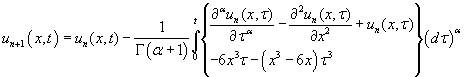(67)

Taking the initial valuewe can derive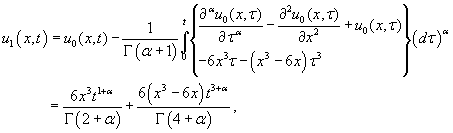(68)

By the similar operations, we have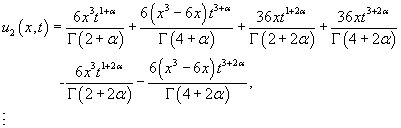(69)

From Eq. (79), variational iteration method for give the following approximations: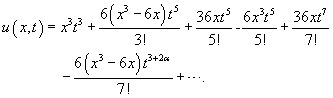(70)

Canceling the noise terms and keeping the non-noise terms in (70) yield the exact solution of Eq. (61), for the special case α = 2 ,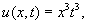(71)

Which is easily verified.

#### Conclusions

The VIM has been successfully applied to derive explicit numerical solutions for nonlinear problems and ordinary, partial, fractional, integral equations. In this paper, we have discussed modified variational iteration method having integral w.r.t.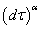used for the first time by Jumarie. The obtained results indicate that this method is powerful and meaningful for solving the nonlinear fractional differential equations. Six examples indicate that the results of variational iteration method having integral w.r.t.are in excellent agreement with those obtained by classical VIM, GDTM, HPM and Adomian decomposition method which is available in the literature.

#### References

Select your language of interest to view the total content in your interested language

### Article Usage

• Total views: 12158
• [From(publication date):
September-2012 - Sep 22, 2019]
• Breakdown by view type
• HTML page views : 8338

## Post your commentCan't read the image? click here to refresh
###### Peer Reviewed Journals

Make the best use of Scientific Research and information from our 700 + peer reviewed, Open Access Journals

International Conferences 2019-20

Meet Inspiring Speakers and Experts at our 3000+ Global Annual Meetings

Top